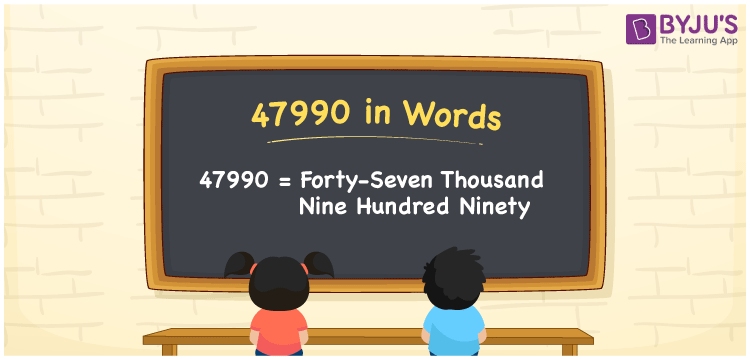# 47990 in words

47990 in words is written as Forty Seven Thousand Nine Hundred and Ninety. In 47990, 4 has a place value of ten thousand, 7 is in the place value of thousand, first 9 has the place value of hundred and the next 9 is in the place value of ten. The article on Place Value gives more information. The number 47990 is used in expressions that relate to money, distance, length, social media views, and many more. For example, “The rent for the conventional hall for a day is Forty Seven Thousand Nine Hundred and Ninety rupees”

 47990 in words Forty Seven Thousand Nine Hundred and Ninety Forty Seven Thousand Nine Hundred and Ninety in Numbers 47990

## 47990 in English Words## How to Write 47990 in Words?

We can convert 47990 to words using a place value chart. This can be done as follows. The number 47990 has 5 digits, so let’s make a chart that shows the place value up to 5 digits.

 Ten thousand Thousands Hundreds Tens Ones 4 7 9 9 0

Thus, we can write the expanded form as:

4 × Ten thousand + 7 × Thousand + 9 × Hundred + 9 × Ten + 0 × One

= 4 × 10000 + 7 × 1000 + 9 × 100 + 9 × 10 + 0 × 1

= 47990.

= Forty Seven Thousand Nine Hundred and Ninety.

47990 is the natural number that is succeeded by 47989 and preceded by 47991.

47990 in words – Forty Seven Thousand Nine Hundred and Ninety.

Is 47990 an odd number? – No.

Is 47990 an even number? – Yes.

Is 47990 a perfect square number? – No.

Is 47990 a perfect cube number? – No.

Is 47990 a prime number? – No.

Is 47990 a composite number? – Yes.

## Solved Example

1. Write the number 47990 in expanded form

Solution: 4 x 10000 + 7 x 1000 + 9 x 100 + 9 x 10 + 0 x 1

Or Just 4 x 10000 + 7 x 1000 + 9 x 100 + 9 x 10

We can write 47990 = 40000 + 7000 + 900 + 90 + 0

= 4 x 10000 + 7 x 1000 + 9 x 100 + 9 x 10 + 0 x 1.

## Frequently Asked Questions on 47990 in words

Q1

### How to write the number 47990 in words?

47990 in words is written as Forty Seven Thousand Nine Hundred and Ninety.
Q2

### Is 47990 divisible by 3?

No. 47990 is not divisible by 3.
Q3

### Is 47990 a prime number?

No. 47990 is not a prime number.